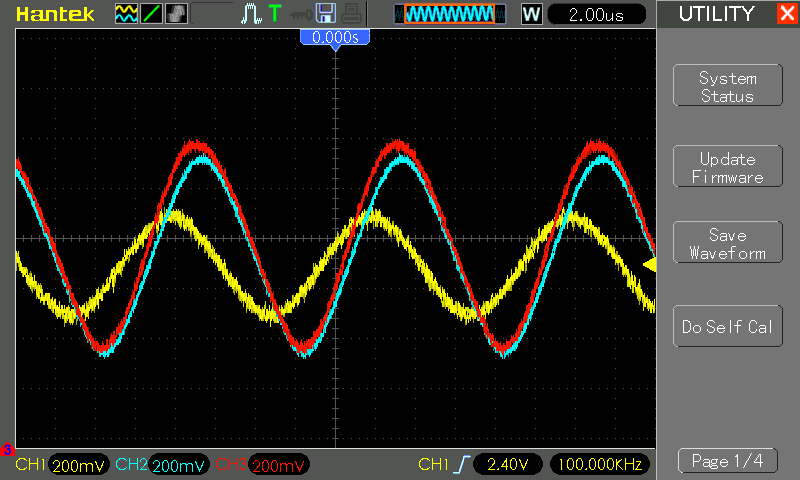# #161 ML741/NonInvertingAmplifier

Test a non-inverting amplifier circuit using the ML741 discrete component opamp

This is a demonstration of a non-inverting amplifier circuit using the ML741 discrete component opamp.

The non-inverting amplifier has an arbitrary gain determined by the input and feedback resistor selection:

``````Vout = (1 + R2/R1) * Vin
``````### How it works

Fundamentally, an op-amp strives to keep its inverting an non-inverting inputs equal by modulating the output.

In the non-inverting amplifier configuration, the non-inverting input is at the inflexion point of the R1:R2 voltage divider. Since the inflexion point must equal the non-inverting input voltage, as the non-inverting input voltage changes, the output voltage must change proportional to R1:R2 in order to maintain equilibrium.

## Construction

The circuit diagrams are drawn with a standard 741 DIP8 package for both the UA741CN and “ML741”. See the ML741 project for details of the actual construction of the ML741.

Both op-amps are configured for a gain of approximtely 3.

### Single-supply Configuration

In this circuit, I am using a single rail supply (V- = GND) instead of the “conventional” dual rail supply (V+/V-). For this reason, the “ground” end of R1 is pegged to V+/2 with a voltage divider. In a dual rail configuration, V+/2 is usually “ground”.

The voltage divider is high impedance, to minimise its interaction with the amplifier circuit. It is also stabilised with a 100nF capacitor.

To improve the isolation of the V+/2 supply, it uses 1/4 of the LM324 as a voltage follower/buffer. The buffer may be omitted at the expense of some gain and DC offset (depending upon frequency).

Without the buffer and capacitor, a simple voltage divider resonates with the circuit and cannot provide a stable supply. Gain will tends towards 1

### Input Buffer

An LM324 is used as an input buffer/splitter so that the ML741 and a standard 741 can be compared at the same time.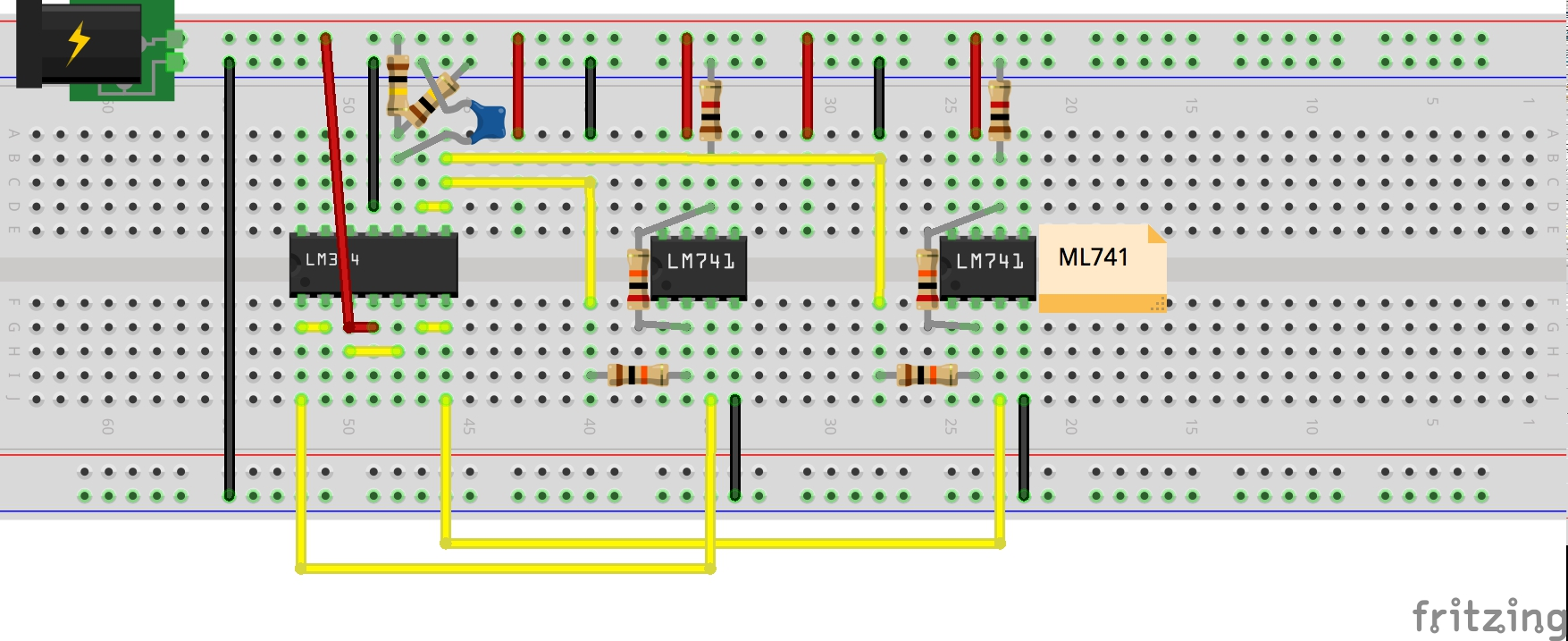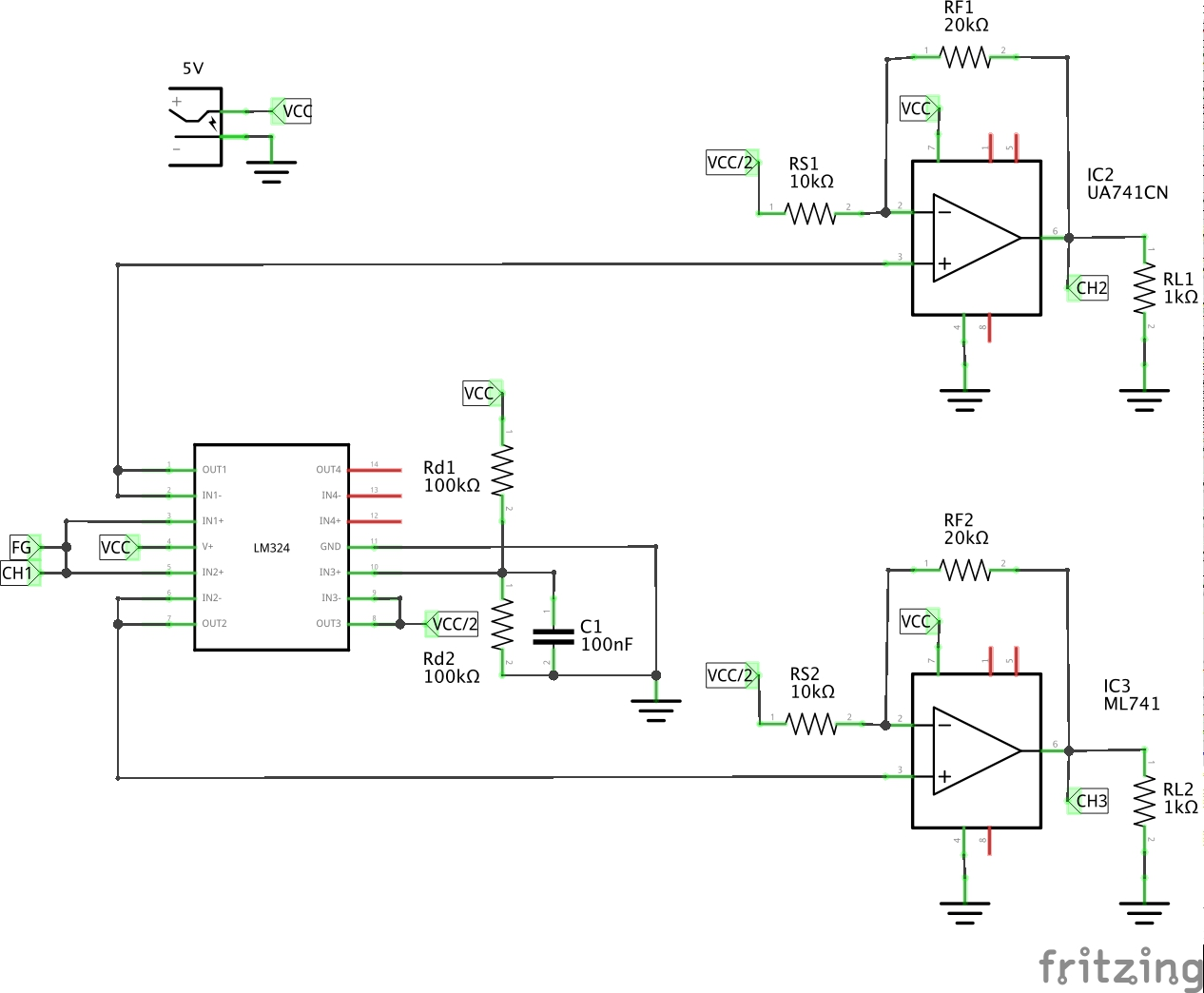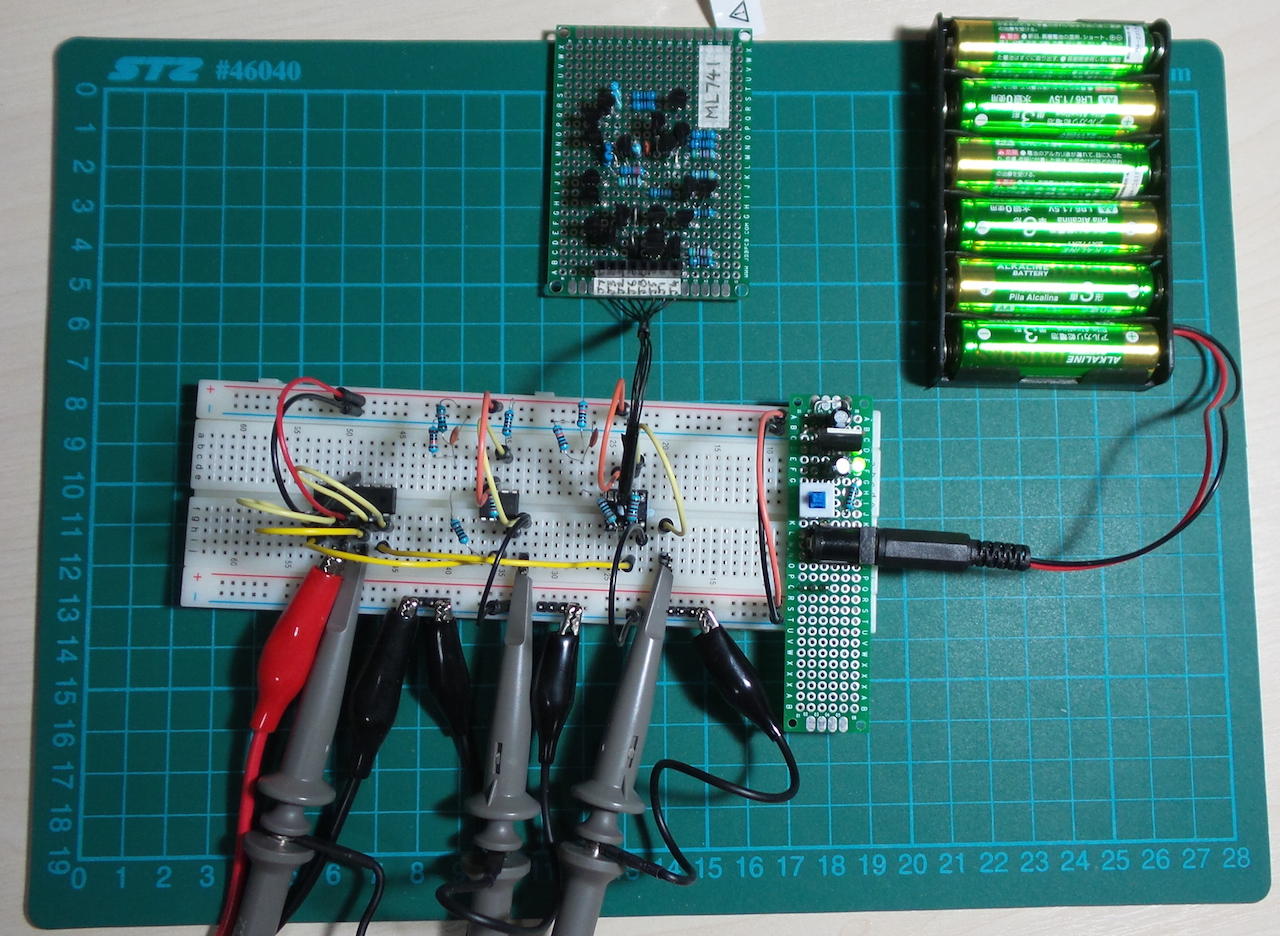## ML741 v “real” 741 Test

Here are some results comparing the behaviour of a standard UA741CN chip with the ML741 (protoboard version).

Setup:

• power is 5V single rail, i.e. V- = GND
• input is a sine wave 200mVpp with 2.5V DC offset, connected at node “FG”
• CH1,CH2 and CH2 signals are DC coupled and vertically shifted by -2.5V

Scope connections

• CH1: input (yellow)
• CH2: UA741CN output (blue)
• CH3: ML741 output (red)

### At 1kHz

• both the ML741 and UA741CN (the real 741) are performing similarly, delivering a gain of just under 3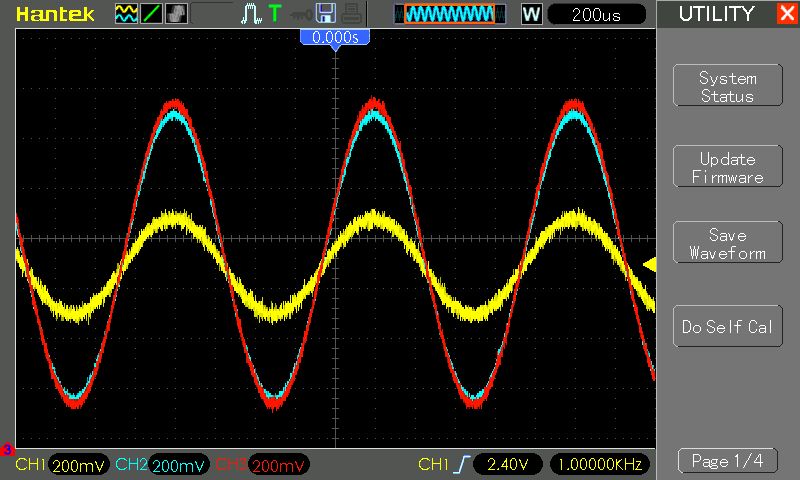### At 100kHz

• gain is being attenutated already, to a similar degree for both the ML741 and UA741CN (the real 741)
• the UA741CN output is phase shifting more than the ML741